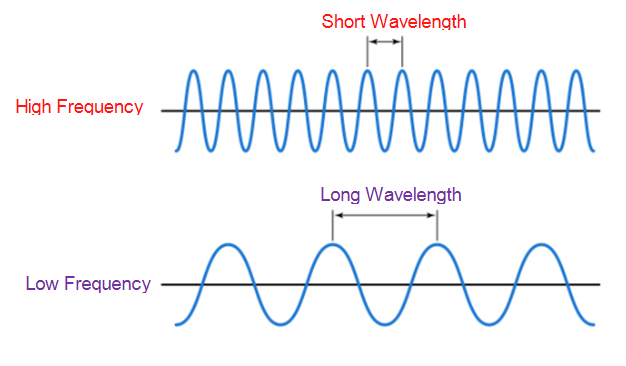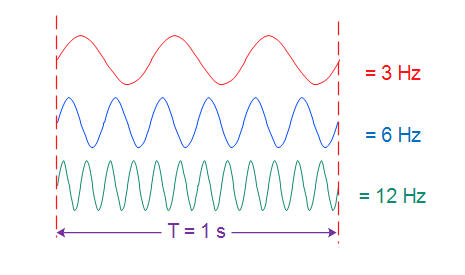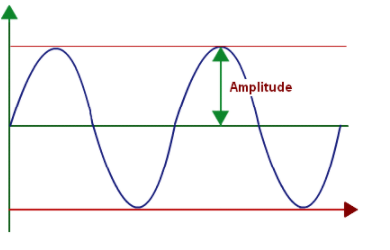# Wavelength, Frequency, Amplitude and phase – defining Waves !

Microwave engineers frequently refers to electromagnetic waves or simply waves and their properties like wavelength, frequency, amplitude and phase.

Wave propagation direction is perpendicular to the direction of forces that creates it. Here forces are electric field and magnetic field.

Electric field is perpendicular to the magnetic field and also is perpendicular to the wave direction. It is like, electric field, magnetic field and wave propagation direction are all perpendicular to each other.#### Wavelength

Wavelength (λ) is the physical length from one point of a wave to the same point on the next wave.Long Wavelength = Low frequency

Short Wavelength = high frequency (more waves in the same time frameIn free space, Speed of light = 3 * 10^8 m/s

Suppose frequency = 2500 MHz

Then, Wavelength = (3 *10^8) / (2000 *10^6)

= 0.12 meter

#### Frequency

The frequency of a wave is the number of waves that pass by each second, and is measured in Hertz (Hz).

Suppose a wave repeat itself 3 times each second, then we say frequency of that wave is 3 Hz.#### AmplitudeAmplitude is the height of the wave and often related to power.

High electric field = High magnetic field = High amplitude = High power

#### Phase

Phase is not a property of just one RF signal but instead involves the relationship between two or more signals that share the same frequency. The phase involves the relationship between the position of the amplitude crests and troughs of two waveforms.

Phase can be measured in distance, time, or degrees. If the peaks of two signals with the same frequency are in exact alignment at the same time, they are said to be in phase. Conversely, if the peaks of two signals with the same frequency are not in exact alignment at the same time, they are said to be out of phase.”This above figure shows two waves 90* out of phase.